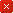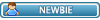Thread: H&R on incompleted set View Single Post
#5TedmorrisSenior MemberJoin Date: Sep 2017 Posts: 61The orignal mod as im sure you know uses snatched mod which works great however the H&R mod was never designed to time stamp seedtime for incompleted torrent sets as the user will always be listed as a leecher due to the torrent not being completed.

PHP Code:
``` \$announcetime = (\$self["seeder"] == "yes" ? "seedtime = seedtime + \$self[announcetime]" : "leechtime = leechtime + \$self[announcetime]");  ```
I think \$announcetime would need to be modified otherwise its just going to add leechtime.

PHP Code:
```  if (isset(\$self) && \$event == "stopped") {\$seeded = 'no';mysql_query("DELETE FROM peers WHERE \$selfwhere") or err("D Err"); //===09 sir_snuggles hit and run \$res_snatch = mysql_query("SELECT seedtime, uploaded, downloaded, finished, start_date AS start_snatch FROM snatched WHERE torrentid = \$torrentid AND userid = {\$user['id']}") or err('Snatch Error 1'); \$a = mysql_fetch_array(\$res_snatch); //=== only run the function if the ratio is below 1 if( (\$a['uploaded'] + \$upthis) < (\$a['downloaded'] + \$downthis) && \$a['finished'] == 'yes') { \$HnR_time_seeded = (\$a['seedtime'] + \$self['announcetime']); //=== get times per class switch (true) {  //=== user case (\$user['class'] < UC_POWER_USER): \$days_3 = 2*86400; //== 2 days \$days_14 = 2*86400; //== 2 days \$days_over_14 = 86400; //== 1 day break; //=== poweruser case (\$user['class'] == UC_POWER_USER): \$days_3 = 129600; //== 36 hours \$days_14 = 129600; //== 36 hours \$days_over_14 = 64800; //== 18 hours break; //=== vip / donor? case (\$user['class'] == UC_VIP): \$days_3 = 129600; //== 36 hours \$days_14 = 86400; //== 24 hours \$days_over_14 = 43200; //== 12 hours break; //=== uploader / staff and above (we don't need this for uploaders now do we? case (\$user['class'] >= UC_UPLOADER): \$days_3 = 43200; //== 12 hours \$days_14 = 43200; //== 12 hours \$days_over_14 = 43200; //== 12 hours break; } switch(true)  { case ((\$a['start_snatch'] - \$torrent['ts']) < 7*86400): \$minus_ratio = (\$days_3 - \$HnR_time_seeded); break; case ((\$a['start_snatch'] - \$torrent['ts']) < 21*86400): \$minus_ratio = (\$days_14 - \$HnR_time_seeded); break; case ((\$a['start_snatch'] - \$torrent['ts']) >= 21*86400): \$minus_ratio = (\$days_over_14 - \$HnR_time_seeded); break; } \$hit_and_run = ((\$minus_ratio > 0 && (\$a['uploaded'] + \$upthis) < (\$a['downloaded'] + \$downthis)) ? ", seeder='no', hit_and_run= '".TIME_NOW."'" : ", hit_and_run = '0'"); } //=== end if not 1:1 ratio else \$hit_and_run = ", hit_and_run = '0'"; //=== end hit and run if (mysql_affected_rows()) { \$updateset[] = (\$self["seeder"] == "yes" ? "seeders = seeders - 1" : "leechers = leechers - 1"); mysql_query("UPDATE snatched SET ip = ".sqlesc(\$ip).", port = \$port, connectable = '\$connectable', uploaded = uploaded + \$upthis, downloaded = downloaded + \$downthis, to_go = \$left, upspeed = \$upspeed, downspeed = \$downspeed, \$announcetime, last_action = ".TIME_NOW.", seeder = '\$seeder', agent = ".sqlesc(\$agent)." \$hit_and_run WHERE torrentid = \$torrentid AND userid = {\$user['id']}") or err("SL Err 1"); }  ```